Write coordinates in brackets.

Write the x coordinate first, then a comma, then the y coordinate.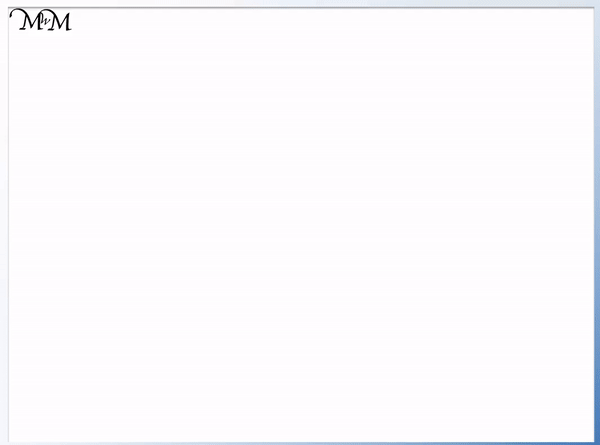• A pair of coordinates are two numbers that tell us the location of a point on a grid.
• Coordinates are two numbers written in between brackets, separated by a comma.
• The first number is called the x-coordinate and tells us how far to the right our point is.
• The second number is called the y-coordinate and tells us how far up our point is.
• To help us remember the order we can say ‘along the corridor, up the stairs’.
• This helps us remember that the first number tells us how far along (right) and the second number tells us how far up.
• Coordinates must be written in this order.• The coordinates of this point are (8, 5).
• The first number tells us how far to the right the point is.
• The 8 tells us the point is 8 right.
• The second number tells us how far up the point is.
• To 5 tells us the point is 5 up.

The first number tells us how far to the right the point is and the second number tells us how far up the point is.

If the first number is negative, the point is to the left. If the second number is negative, the point is downwards.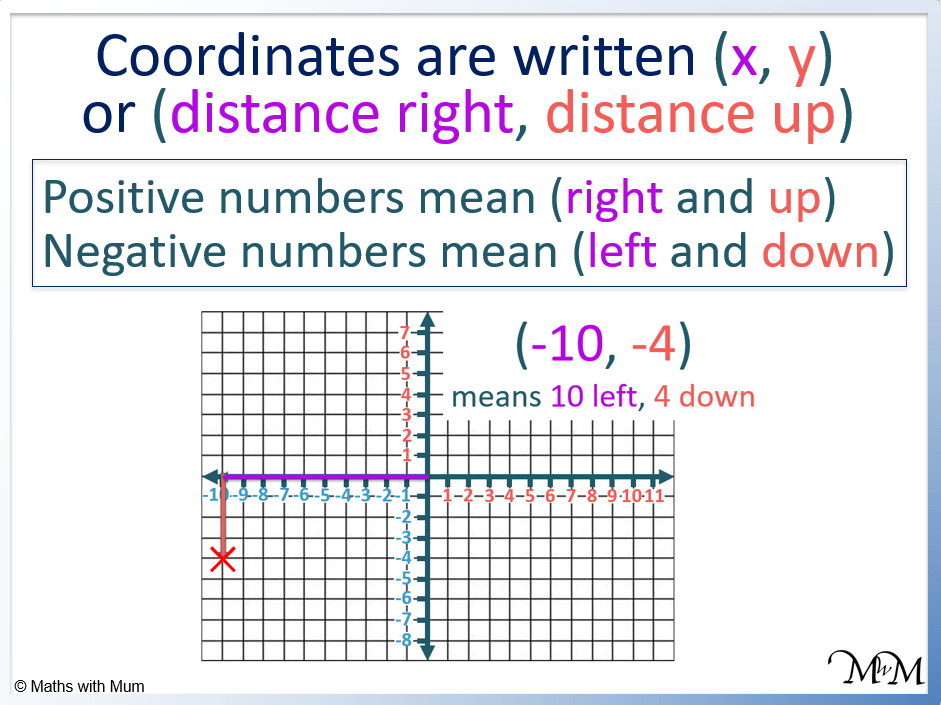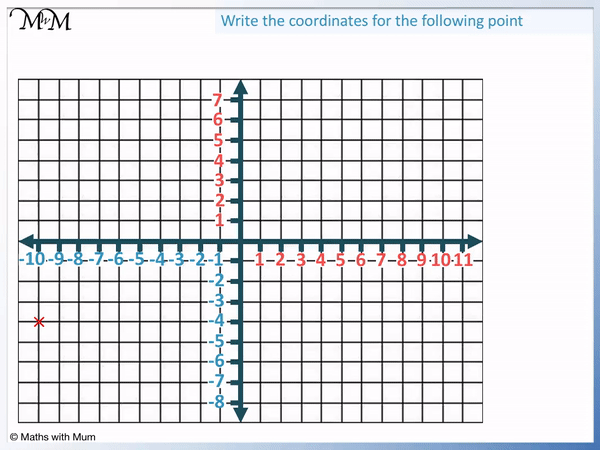• A positive x coordinate tells us that the point is to the right.
• A negative x coordinate indicates that the point is to the left.
• A positive y coordinate indicates that the point is upwards.
• A negative y coordinate indicates that the point is downwards.
• The coordinate pair (-10, -4) has an x coordinate of -10 and a y coordinate of -4.
• The point is found 10 left and 4 down.#### How to Read Coordinates on a Grid: Video LessonCoordinates are 2 numbers written in brackets, separated by a comma. The first number is the x coordinate which when positive, indicates how far right the point is and when negative, indicates how far left the point is. The second number is the y coordinate which when positive, indicates how far up the point is and when negative, indicates how far down the point is.

The order in which coordinates are written matters. The first number is the x coordinate and the second number is the y coordinate.

For example, the coordinate (4, 9) has an x coordinate of 4 and a y coordinate of 9.The x coordinate is 4. This is a positive number and so, it means to move 4 places right. This means we move 4 places right from where the axes cross over at (0, 0).

The y coordinate is 9. This means that after moving 4 places right, we move 9 places up. We know that we move upwards because 9 is a positive number.To remember the order that the first number tells us to go right and the second number tells us to go up, we use the phrase ‘along the corridor, up the stairs’.

The ‘along the corridor’ represents us going along (to the right) and the ‘up the stairs’ represents going up.

This phrase is commonly used in schools when teaching coordinates because it helps to avoid the most common mistake of writing the two numbers in the wrong order.

To read coordinates from a grid, compare the position of the coordinate to the origin where the axes meet. First write the number of places the coordinate is to the right of the y-axis. If it is to the left, this number is negative. Now write a comma and then write the number of places the coordinate is above the x-axis. If it is below, this number is negative.Coordinates are always measured from the origin. The origin is where the x and y axes cross over at the centre of the grid.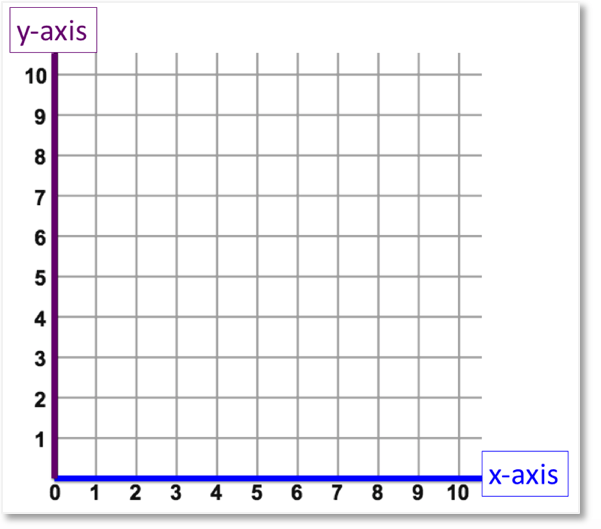We compare the position of the coordinate to the origin to read the coordinate.

• Right is positive.
• Left is negative.
• Up is positive.
• Down is negative.

The point is 10 left of the origin. Since it is left, we write down -10. Negative x coordinates mean left.

The point is 4 below the origin. Since it is down, we write -4. Negative y coordinates mean down.The coordinates are written (-10, -4). The numbers must be written in this order.

Write the x coordinate first, which is how far left or right the coordinate is. Then write the y coordinate second, which is how far up or down the coordinate is.

## How to Plot Coordinates

To plot coordinates:

1. Read the first coordinate before the comma.
2. Along the x-axis, move this many places right if it is positive or left if it is negative.
3. Read the second coordinate after the comma.
4. Move this many places up if the number is positive or down if it is negative.
5. Draw a cross at this position.

For example, plot (-8, 2).

The first number, the x coordinate, is -8. It is negative, so we move 8 places left.

Now we read the second number, the y coordinate, which is 2. It is positive so we move 2 places up.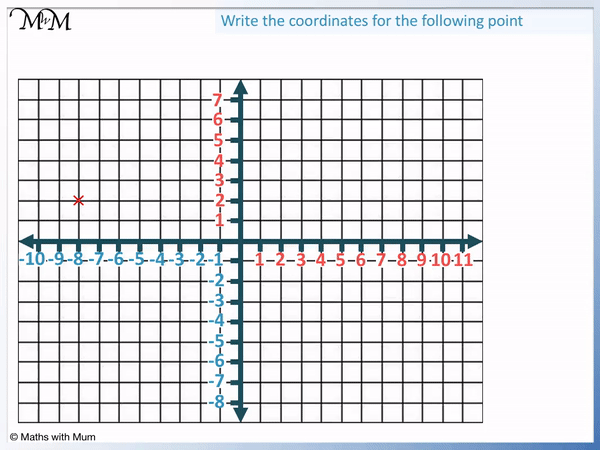For example, plot the coordinate (6, -6).The x coordinate is positive, so we move 6 places right.

The y coordinate is negative, so we move 6 places down.

For example, plot (3, 5).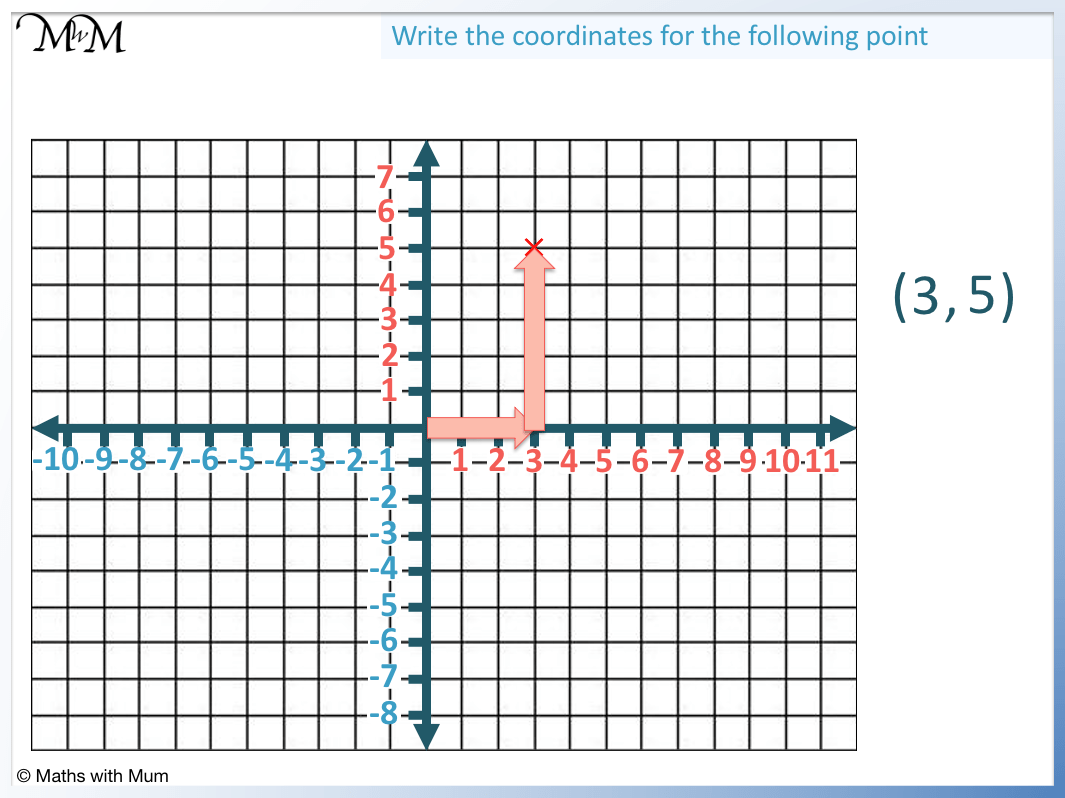The x coordinate is 3, so we move 3 places right.

The y coordinate is 5, so we move 5 places up.

## The Order of Coordinates Matters

The order in which coordinates are written matters. The x coordinate must be written first and the y coordinate must be written second. The x coordinate describes the horizontal position and the y coordinate describes the vertical position. If the x and y coordinates are written in the wrong order, the coordinate will be describing the wrong position.

For example:

The coordinate (1, 2) means 1 to the right and 2 up.

The coordinate (2, 1) means 2 to the right and 1 up.We can see that switching the order of the coordinates results in the point being located in a different position.

When learning how to write coordinates, the most common mistake is to write the x and y coordinates in the wrong order.

Use the phrase, ‘along the corridor and up the stairs’ to remember that the first coordinate describes how far along the point is and the second coordinate describes how far up or down the point is.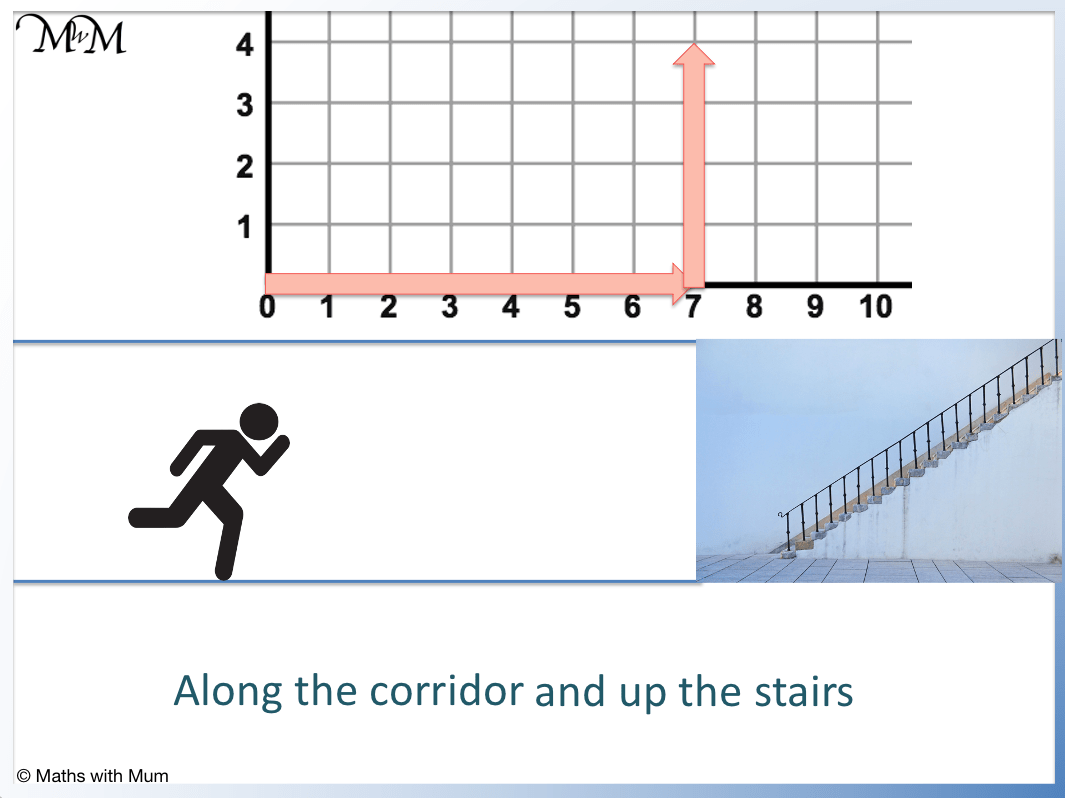## Reading Coordinates with Fractions and Decimals

Coordinates can be located at any point on the grid, including inside the squares of the grid. If a coordinate is located directly between two whole numbers, it will contain fractions of one half. If the coordinate is not directly in between two whole numbers, use the scale to estimate the location as a decimal.

For example, here is the coordinate (4   1/2, 3   1/2).

The coordinate is located directly between 4 and 5 on the x axis and so has an x coordinate of 4   1/2.

The coordinate is located directly between 3 and 4 on the y axis and so has a y coordinate of 3   1/2.Here is an example of a coordinate that is not in the centre of a grid and so, it is nearer to one number than the other.

Here we estimate its position and we can write it as a decimal.The coordinate is very close to 2 on the x axis but it is not quite on 2. It is at 1.9.

The coordinate is very close to 1 on the y axis but it is just above 1. It is at 1.1.

## How to Read 3D Coordinates

To read coordinates in 3D, read the distance travelled in the direction of the x axis, y axis and z axis in that order. There are 3 numbers that make up a set of coordinates in 3D and they are always written in the form (x, y, z).

For example, Here is the point (1, 3, 2).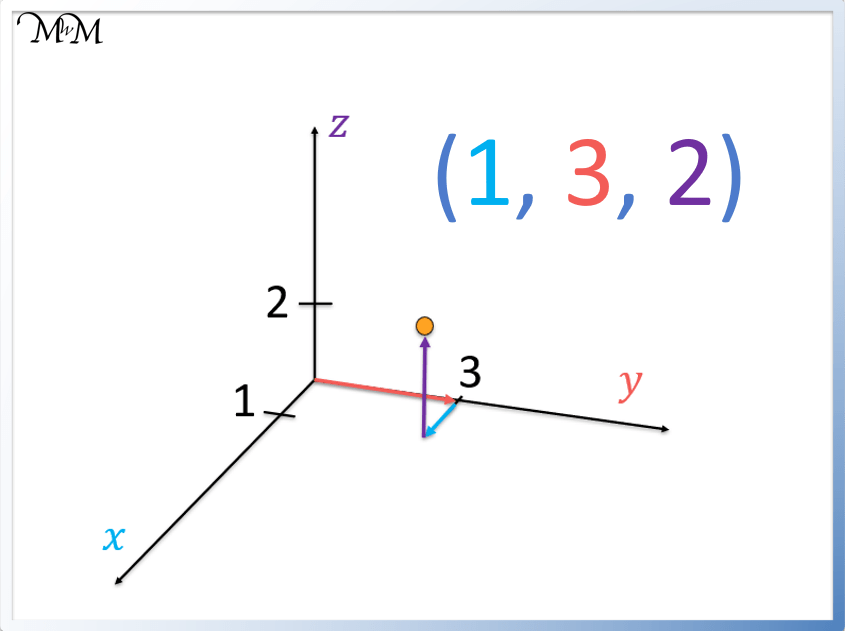The point has an x coordinate of 1, a y coordinate of 3 and a z coordinate of 2.

Here is the 3D coordinate of (2, 0, 5).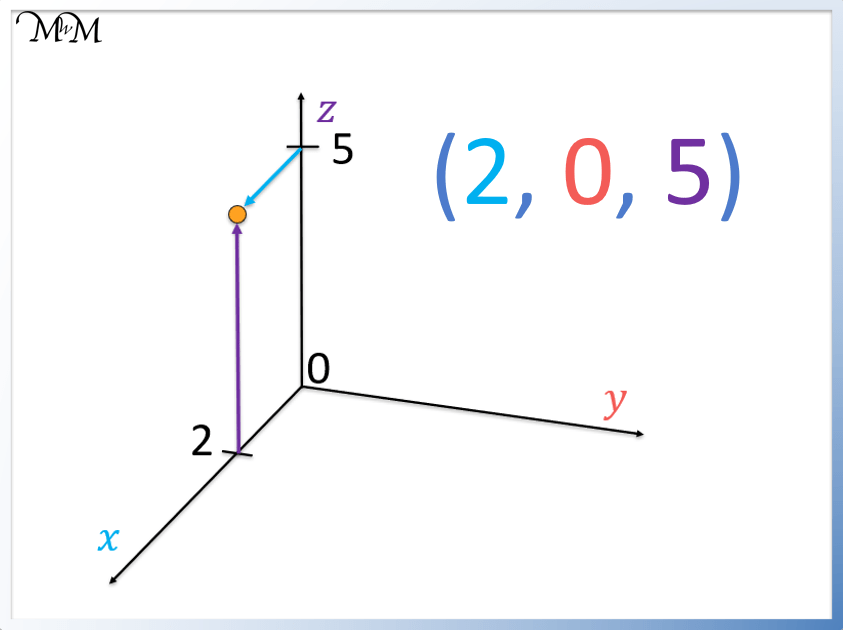The point has an x coordinate of 2, a y coordinate of 0 and a z coordinate of 5.Now try our lesson on Reflecting Shapes where we learn how to reflect a shape on a grid.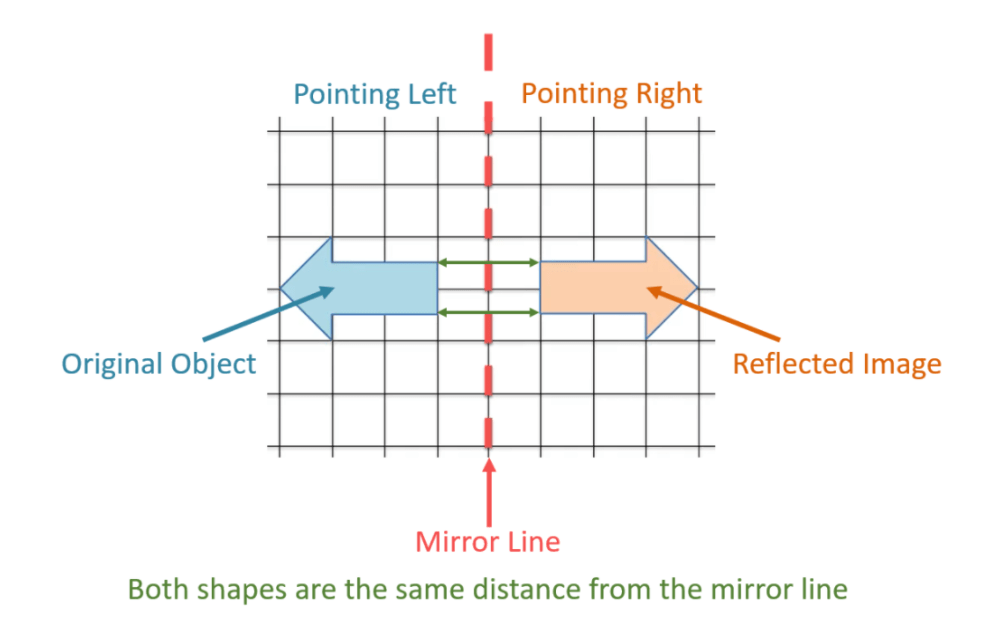error: Content is protected !!